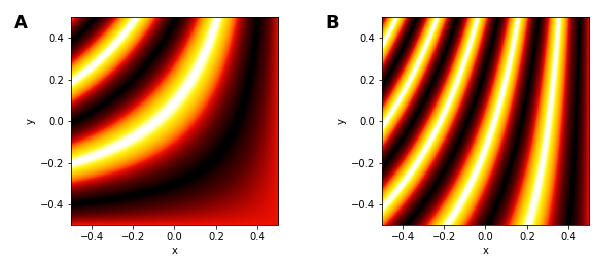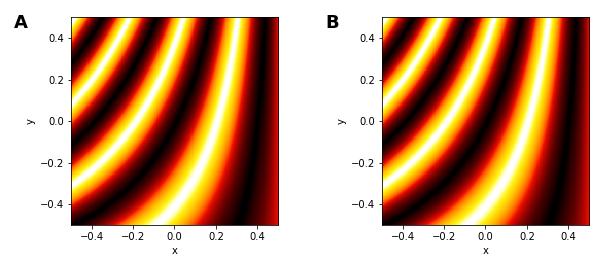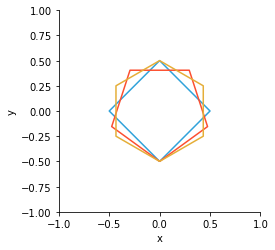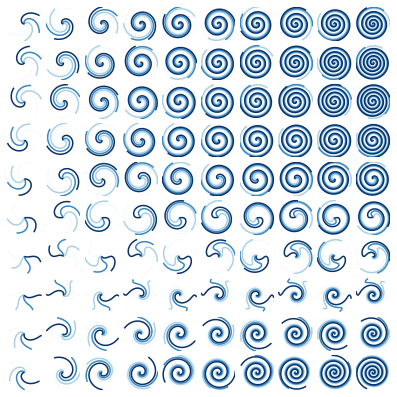# Live Data¶

The HoloMap is a core HoloViews data structure that allows easy exploration of parameter spaces. The essence of a HoloMap is that it contains a collection of Elements (e.g. Images and Curves) that you can easily select and visualize.

HoloMaps hold fully constructed Elements at specifically sampled points in a multidimensional space. Although HoloMaps are useful for exploring high-dimensional parameter spaces, they can very quickly consume huge amounts of memory to store all these Elements. For instance, a hundred samples along four orthogonal dimensions would need a HoloMap containing a hundred million Elements, each of which could be a substantial object that takes time to create and costs memory to store. Thus HoloMaps have some clear limitations:

• HoloMaps may require the generation of millions of Elements before the first element can be viewed.
• HoloMaps can easily exhaust all the memory available to Python.
• HoloMaps can even more easily exhaust all the memory in the browser when displayed.
• Static export of a notebook containing HoloMaps can result in impractically large HTML files.

The DynamicMap addresses these issues by computing and displaying elements dynamically, allowing exploration of much larger datasets:

• DynamicMaps generate elements on the fly, allowing the process of exploration to begin immediately.
• DynamicMaps do not require fixed sampling, allowing exploration of parameters with arbitrary resolution.
• DynamicMaps are lazy in the sense they only compute as much data as the user wishes to explore.

Of course, these advantages come with some limitations:

• DynamicMaps require a live notebook server and cannot be fully exported to static HTML.
• DynamicMaps store only a portion of the underlying data, in the form of an Element cache and their output is dependent on the particular version of the executed code.
• DynamicMaps (and particularly their element caches) are typically stateful (with values that depend on patterns of user interaction), which can make them more difficult to reason about.

In addition to the different computational requirements of DynamicMaps, they can be used to build sophisticated, interactive vizualisations that cannot be achieved using only HoloMaps. This notebook demonstrates some basic examples and the Responding to Events guide follows on by introducing the streams system. The Custom Interactivity shows how you can directly interact with your plots when using the Bokeh backend.

When DynamicMap was introduced in version 1.6, it supported multiple different 'modes' which have now been deprecated. This notebook demonstrates the simpler, more flexible and more powerful DynamicMap introduced in version 1.7. Users who have been using the previous version of DynamicMap should be unaffected as backwards compatibility has been preserved for the most common cases.

All this will make much more sense once we've tried out some DynamicMaps and showed how they work, so let's create one!

## DynamicMap¶

In :
import numpy as np
import holoviews as hv
from holoviews import opts

hv.extension('matplotlib')We will now create DynamicMap similar to the HoloMap introduced in the Introductory guide. The HoloMap in that introduction consisted of Image elements defined by a function returning NumPy arrays called sine_array. Here we will define a waves_image function that returns an array pattern parameterized by arbitrary alpha and beta parameters inside a HoloViews Image element:

In :
xvals  = np.linspace(-4, 0, 202)
yvals  = np.linspace(4, 0, 202)
xs, ys = np.meshgrid(xvals, yvals)

def waves_image(alpha, beta):
return hv.Image(np.sin(((ys/alpha)**alpha+beta)*xs))

waves_image(1,0) + waves_image(1,4)

Out:Now we can demonstrate the possibilities for exploration enabled by the simplest declaration of a DynamicMap.

### Basic DynamicMap declaration¶

A simple DynamicMap declaration looks identical to that needed to declare a HoloMap. Instead of supplying some initial data, we will supply the waves_image function with key dimensions simply declaring the arguments of that function:

In :
dmap = hv.DynamicMap(waves_image, kdims=['alpha', 'beta'])
dmap

DynamicMap cannot be displayed without explicit indexing as 'alpha', 'beta' dimension(s) are unbounded.
Set dimensions bounds with the DynamicMap redim.range or redim.values methods.
Out:
:DynamicMap   [alpha,beta]

This object is created instantly, but because it doesn't generate any hv.Image objects initially it only shows the printed representation of this object along with some information about how to display it. We will refer to a DynamicMap that doesn't have enough information to display itself as 'unbounded'.

The textual representation of all DynamicMaps look similar, differing only in the listed dimensions until they have been evaluated at least once.

#### Explicit indexing¶

Unlike a corresponding HoloMap declaration, this simple unbounded DynamicMap cannot yet visualize itself. To view it, we can follow the advice in the warning message. First we will explicitly index into our DynamicMap in the same way you would access a key on a HoloMap:

In :
dmap[1,2] + dmap.select(alpha=1, beta=2)

Out:Note that the declared kdims are specifying the arguments by position as they do not match the argument names of the waves_image function. If you do match the argument names exactly, you can map a kdim position to any argument position of the callable. For instance, the declaration kdims=['freq', 'phase'] would index first by frequency, then phase without mixing up the arguments to waves_image when indexing.

#### Setting dimension ranges¶

The second suggestion in the warning message was to supply dimension ranges using the redim.range method:

In :
dmap.redim.range(alpha=(1, 5.0), beta=(1, 6.0))

Out:

Here each hv.Image object visualizing a particular sine ring pattern with the given parameters is created dynamically, whenever the slider is set to a new value. Any value in the allowable range can be requested by dragging the sliders or by tweaking the values using the left and right arrow keys.

Of course, we didn't have to use the redim.range method and we could have simply declared the ranges right away using explicit hv.Dimension objects. This would allow us to declare other dimension properties such as the step size used by the sliders: by default each slider can select around a thousand distinct values along its range but you can specify your own step value via the dimension step parameter. If you use integers in your range declarations, integer stepping will be assumed with a step size of one.

Note that whenever the redim method is used, a new DynamicMap is returned with the updated dimensions. In other words, the original dmap remains unbounded with default dimension objects.

#### Setting dimension values¶

The DynamicMap above allows exploration of any phase and frequency within the declared range unlike an equivalent HoloMap which would have to be composed of a finite set of samples. We can achieve a similar discrete sampling using DynamicMap by setting the values parameter on the dimensions:

In :
dmap.redim.values(alpha=[1, 2, 3], beta=[0.1, 1.0, 2.5])

Out:

The sliders now snap to the specified dimension values and if you are running this live, the above cell should look like a HoloMap. DynamicMap is in fact a subclass of HoloMap with some crucial differences:

• You can now pick as many values of alpha or beta as allowed by the slider.
• What you see in the cell above will not be exported in any HTML snapshot of the notebook

We will now explore how DynamicMaps relate to HoloMaps including conversion operations between the two types. As we will see, there are other ways to display a DynamicMap without using explicit indexing or redim.

## Interaction with HoloMaps¶

To explore the relationship between DynamicMap and HoloMap, let's declare another callable to draw some shapes we will use in a new DynamicMap:

In :
def shapes(N, radius=0.5): # Positional keyword arguments are fine
for a in np.linspace(-np.pi, np.pi, n+2)]],
extents=(-1,-1,1,1))
for n in range(N,N+3)]
return hv.Overlay(paths)


#### Sampling DynamicMap from a HoloMap¶

When combining a HoloMap with a DynamicMap, it would be very awkward to have to match the declared dimension values of the DynamicMap with the keys of the HoloMap. Fortunately you don't have to:

In :
holomap = hv.HoloMap({(N,r):shapes(N, r) for N in [3,4,5] for r in [0.5,0.75]},  kdims=['N', 'radius'])
holomap + dmap

Out:

Here we declared a DynamicMap without using redim, but we can view its output because it is presented alongside a HoloMap which defines the available keys. This convenience is subject to three particular restrictions:

• You cannot display a layout consisting of unbounded DynamicMaps only, because at least one HoloMap is needed to define the samples.
• The HoloMaps provide the necessary information required to sample the DynamicMap.

Note that there is one way DynamicMap is less restricted than HoloMap: you can freely combine bounded DynamicMaps together in a Layout, even if they don't share key dimensions.

#### Converting from DynamicMap to HoloMap¶

Above we mentioned that DynamicMap is an instance of HoloMap. Does this mean it has a .data attribute?

In :
dtype = type(dmap.data).__name__
length = len(dmap.data)
print("DynamicMap 'dmap' has an {dtype} .data attribute of length {length}".format(dtype=dtype, length=length))

DynamicMap 'dmap' has an OrderedDict .data attribute of length 1


This is exactly the same sort of .data as the equivalent HoloMap, except that its values will vary according to how much you explored the parameter space of dmap using the sliders above. In a HoloMap, .data contains a defined sampling along the different dimensions, whereas in a DynamicMap, the .data is simply the cache.

The cache serves two purposes:

• Avoids recomputation of an element should we revisit a particular point in the parameter space. This works well for categorical or integer dimensions, but doesn't help much when using continuous sliders for real-valued dimensions.
• Records the space that has been explored with the DynamicMap for any later conversion to a HoloMap up to the allowed cache size.

We can always convert any DynamicMap directly to a HoloMap as follows:

In :
hv.HoloMap(dmap)

Out:This is in fact equivalent to declaring a HoloMap with the same parameters (dimensions, etc.) using dmap.data as input, but is more convenient. Note that the slider positions reflect those we sampled from the HoloMap in the previous section.

Although creating a HoloMap this way is easy, the result is poorly controlled, as the keys in the DynamicMap cache are usually defined by how you moved the sliders around. If you instead want to specify a specific set of samples, you can easily do so by using the same key-selection semantics as for a HoloMap to define exactly which elements are to be sampled and put into the cache:

In :
hv.HoloMap(dmap[{(2,0.3), (2,0.6), (3,0.3), (3,0.6)}])

Out:

Here we index the dmap with specified keys to return a new DynamicMap with those keys in its cache, which we then cast to a HoloMap. This allows us to export specific contents of DynamicMap to static HTML which will display the data at the sampled slider positions.

The key selection above happens to define a Cartesian product, which is one of the most common ways to sample across dimensions. Because the list of such dimension values can quickly get very large when enumerated as above, we provide a way to specify a Cartesian product directly, which also works with HoloMaps. Here is an equivalent way of defining the same set of four points in that two-dimensional space:

In :
samples = hv.HoloMap(dmap[{2,3},{0.5,1.0}])
samples

Out:
In :
samples.data.keys()

Out:
odict_keys([(2, 0.5), (2, 1.0), (3, 0.5), (3, 1.0)])

The default cache size of 500 Elements is relatively high so that interactive exploration will work smoothly, but you can reduce it using the cache_size parameter if you find you are running into issues with memory consumption. A bounded DynamicMap with cache_size=1 requires the least memory, but will recompute a new Element every time the sliders are moved, making it less responsive.

#### Converting from HoloMap to DynamicMap¶

We have now seen how to convert from a DynamicMap to a HoloMap for the purposes of static export, but why would you ever want to do the inverse?

Although having a HoloMap to start with means it will not save you memory, converting to a DynamicMap does mean that the rendering process can be deferred until a new slider value requests an update. You can achieve this conversion using the Dynamic utility as demonstrated here by applying it to the previously defined HoloMap called samples:

In :
from holoviews.util import Dynamic
dynamic = Dynamic(samples)
print('After apply Dynamic, the type is a {dtype}'.format(dtype=type(dynamic).__name__))
dynamic

After apply Dynamic, the type is a DynamicMap

Out:

In this particular example, there is no real need to use Dynamic as each frame renders quickly enough. For visualizations that are slow to render, using Dynamic can result in more responsive visualizations.

The Dynamic utility is very versatile and is discussed in more detail in the Transforming Elements guide.

### Slicing DynamicMaps¶

As we have seen we can either declare dimension ranges directly in the kdims or use the redim.range convenience method:

In :
dmap = hv.DynamicMap(shapes, kdims=['N','radius']).redim.range(N=(2,20), radius=(0.5,1.0))


The declared dimension ranges define the absolute limits allowed for exploration in this continuous, bounded DynamicMap . That said, you can use the soft_range parameter to view subregions within that range. Setting the soft_range parameter on dimensions can be done conveniently using slicing:

In :
sliced = dmap[4:8, :]
sliced

Out:

Notice that N is now restricted to the range 4:8. Open slices are used to release any soft_range values, which resets the limits back to those defined by the full range:

In :
sliced[:, 0.8:1.0]

Out:

The [:] slice leaves the soft_range values alone and can be used as a convenient way to clone a DynamicMap. Note that mixing slices with any other object type is not supported. In other words, once you use a single slice, you can only use slices in that indexing operation.

## Using groupby to discretize a DynamicMap¶

A DynamicMap also makes it easy to partially or completely discretize a function to evaluate in a complex plot. By grouping over specific dimensions that define a fixed sampling via the Dimension values parameter, the DynamicMap can be viewed as a GridSpace, NdLayout, or NdOverlay. If a dimension specifies only a continuous range it can't be grouped over, but it may still be explored using the widgets. This means we can plot partial or completely discretized views of a parameter space easily.

#### Partially discretize¶

The implementation for all the groupby operations uses the .groupby method internally, but we also provide three higher-level convenience methods to group dimensions into an NdOverlay (.overlay), GridSpace (.grid), or NdLayout (.layout).

Here we will evaluate a simple sine function with three dimensions, the phase, frequency, and amplitude. We assign the frequency and amplitude discrete samples, while defining a continuous range for the phase:

In :
xs = np.linspace(0, 2*np.pi,100)

def sin(ph, f, amp):
return hv.Curve((xs, np.sin(xs*f+ph)*amp))

kdims=[hv.Dimension('phase', range=(0, np.pi)),
hv.Dimension('frequency', values=[0.1, 1, 2, 5, 10]),
hv.Dimension('amplitude', values=[0.5, 5, 10])]

waves_dmap = hv.DynamicMap(sin, kdims=kdims)


Next we define the amplitude dimension to be overlaid and the frequency dimension to be gridded:

In :
wave_grid = waves_dmap.overlay('amplitude').grid('frequency')
wave_grid.opts(fig_size=200, show_legend=True)

Out:

As you can see, instead of having three sliders (one per dimension), we've now laid out the frequency dimension as a discrete set of values in a grid, and the amplitude dimension as a discrete set of values in an overlay, leaving one slider for the remaining dimension (phase). This approach can help you visualize a large, multi-dimensional space efficiently, with full control over how each dimension is made visible.

#### Fully discretize¶

Given a continuous function defined over a space, we could sample it manually, but here we'll look at an example of evaluating it using the groupby method. Let's look at a spiral function with a frequency and first- and second-order phase terms. Then we define the dimension values for all the parameters and declare the DynamicMap:

In :
opts.defaults(opts.Path(color=hv.Palette('Blues')))

def spiral_equation(f, ph, ph2):
r = np.arange(0, 1, 0.005)
xs, ys = (r * fn(f*np.pi*np.sin(r+ph)+ph2) for fn in (np.cos, np.sin))
return hv.Path((xs, ys))

spiral_dmap = hv.DynamicMap(spiral_equation, kdims=['f','ph','ph2'])
spiral_dmap = spiral_dmap.redim.values(
f=np.linspace(1, 10, 10),
ph=np.linspace(0, np.pi, 10),
ph2=np.linspace(0, np.pi, 4))


Now we can make use of the .groupby method to group over the frequency and phase dimensions, which we will display as part of a GridSpace by setting the container_type. This leaves the second phase variable, which we assign to an NdOverlay by setting the group_type:

In :
spiral_grid = spiral_dmap.groupby(['f', 'ph'], group_type=hv.NdOverlay, container_type=hv.GridSpace)
spiral_grid.opts(
opts.GridSpace(xaxis=None, yaxis=None),
opts.Path(bgcolor='white', xaxis=None, yaxis=None))

Out:This grid shows a range of frequencies f on the x axis, a range of the first phase variable ph on the y axis, and a range of different ph2 phases as overlays within each location in the grid. As you can see, these techniques can help you visualize multidimensional parameter spaces compactly and conveniently.

## DynamicMaps and normalization¶

By default, a HoloMap normalizes the display of elements using the minimum and maximum values found across the HoloMap. This automatic behavior is not possible in a DynamicMap, where arbitrary new elements are being generated on the fly. Consider the following examples where the arrays contained within the returned Image objects are scaled with time:

In :
ls = np.linspace(0, 10, 200)
xx, yy = np.meshgrid(ls, ls)

def cells(time):
return hv.Image(time*np.sin(xx+time)*np.cos(yy+time), vdims='Intensity')

dmap = hv.DynamicMap(cells, kdims='time').redim.range(time=(1,20))
(dmap + dmap.redim.range(Intensity=(0,10))).opts(
opts.Image(axiswise=True))

Out:

Here we use axiswise=True to see the behavior of the two cases independently. We see in A that when only the time dimension is given a range, no automatic normalization occurs (unlike a HoloMap). In B we see that normalization is applied, but only when the value dimension ('Intensity') range has been specified.

In other words, DynamicMaps cannot support automatic normalization across their elements, but do support the same explicit normalization behavior as HoloMaps. Values that are generated outside this range are simply clipped in accord with the usual semantics of explicit value dimension ranges.

Note that we always have the option of casting a DynamicMap to a HoloMap in order to automatically normalize across the cached values, without needing explicit value dimension ranges.

## Using DynamicMaps in your code¶

As you can see, DynamicMaps let you use HoloViews with a very wide range of dynamic data formats and sources, making it simple to visualize ongoing processes or very large data spaces.

Given unlimited computational resources, the functionality covered in this guide would match that offered by HoloMap but with fewer normalization options. DynamicMap actually enables a vast range of new possibilities for dynamic, interactive visualizations as covered in the Responding to Events guide. Following on from that, the Custom Interactivity guide shows how you can directly interact with your plots when using the Bokeh backend.